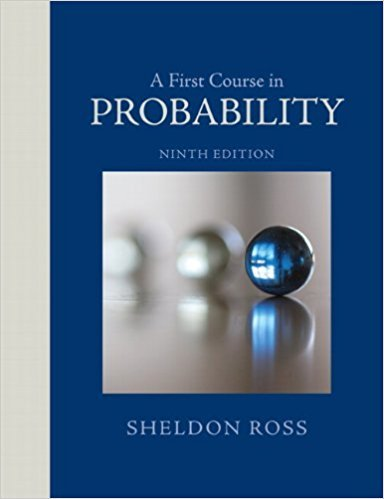×
×

# Solutions for Chapter 4: A First Course in Probability 9th Edition## Full solutions for A First Course in Probability | 9th Edition

ISBN: 9780321794772Solutions for Chapter 4

Solutions for Chapter 4
4 5 0 407 Reviews
19
0
##### ISBN: 9780321794772

Chapter 4 includes 142 full step-by-step solutions. A First Course in Probability was written by and is associated to the ISBN: 9780321794772. Since 142 problems in chapter 4 have been answered, more than 62401 students have viewed full step-by-step solutions from this chapter. This textbook survival guide was created for the textbook: A First Course in Probability , edition: 9. This expansive textbook survival guide covers the following chapters and their solutions.

Key Statistics Terms and definitions covered in this textbook
• Alias

In a fractional factorial experiment when certain factor effects cannot be estimated uniquely, they are said to be aliased.

• All possible (subsets) regressions

A method of variable selection in regression that examines all possible subsets of the candidate regressor variables. Eficient computer algorithms have been developed for implementing all possible regressions

• Analysis of variance (ANOVA)

A method of decomposing the total variability in a set of observations, as measured by the sum of the squares of these observations from their average, into component sums of squares that are associated with speciic deined sources of variation

• Asymptotic relative eficiency (ARE)

Used to compare hypothesis tests. The ARE of one test relative to another is the limiting ratio of the sample sizes necessary to obtain identical error probabilities for the two procedures.

• Biased estimator

Unbiased estimator.

• Conditional probability density function

The probability density function of the conditional probability distribution of a continuous random variable.

• Conidence interval

If it is possible to write a probability statement of the form PL U ( ) ? ? ? ? = ?1 where L and U are functions of only the sample data and ? is a parameter, then the interval between L and U is called a conidence interval (or a 100 1( )% ? ? conidence interval). The interpretation is that a statement that the parameter ? lies in this interval will be true 100 1( )% ? ? of the times that such a statement is made

• Contrast

A linear function of treatment means with coeficients that total zero. A contrast is a summary of treatment means that is of interest in an experiment.

• Cook’s distance

In regression, Cook’s distance is a measure of the inluence of each individual observation on the estimates of the regression model parameters. It expresses the distance that the vector of model parameter estimates with the ith observation removed lies from the vector of model parameter estimates based on all observations. Large values of Cook’s distance indicate that the observation is inluential.

• Covariance

A measure of association between two random variables obtained as the expected value of the product of the two random variables around their means; that is, Cov(X Y, ) [( )( )] =? ? E X Y ? ? X Y .

• Defect concentration diagram

A quality tool that graphically shows the location of defects on a part or in a process.

• Deining relation

A subset of effects in a fractional factorial design that deine the aliases in the design.

• Dependent variable

The response variable in regression or a designed experiment.

• Design matrix

A matrix that provides the tests that are to be conducted in an experiment.

• Distribution function

Another name for a cumulative distribution function.

• Erlang random variable

A continuous random variable that is the sum of a ixed number of independent, exponential random variables.

• Error sum of squares

In analysis of variance, this is the portion of total variability that is due to the random component in the data. It is usually based on replication of observations at certain treatment combinations in the experiment. It is sometimes called the residual sum of squares, although this is really a better term to use only when the sum of squares is based on the remnants of a model-itting process and not on replication.

• Estimate (or point estimate)

The numerical value of a point estimator.

• Estimator (or point estimator)

A procedure for producing an estimate of a parameter of interest. An estimator is usually a function of only sample data values, and when these data values are available, it results in an estimate of the parameter of interest.

• Finite population correction factor

A term in the formula for the variance of a hypergeometric random variable.

×